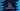# C++ program to implement binary search## Introduction to binary search :

Binary Search uses divide and search approach to search for an element in a sorted array. It divides the searching array into two parts and checks on which part the element lies. Based on that, it moves to either the first part or second part. Then it divides that part and follows the same rule.

Repeatedly, it narrows the interval down and finds the element if it exist.

## Time complexity :

The time complexity of binary search is O(log n).

In this post, I will show you how to implement binary search in C++. I will show you both recursive and iterative way to solve it.

## Recursive method :

The below code implements binary search recursively :

``````using namespace std;
#include<iostream>

int findUsingBinarySearch(int arr[], int numToFind, int left, int right)
{

if (right >= left)
{
int midPosition = left + (right - left) / 2;

if (arr[midPosition] == numToFind)
return midPosition;

if (numToFind < arr[midPosition])
return findUsingBinarySearch(arr, numToFind, left, midPosition - 1s);

return findUsingBinarySearch(arr, numToFind, midPosition + 1, right);
}

return -1;
}

int main(void)
{
int givenArray[] = {1, 5, 7, 8, 10, 20, 28, 30, 40, 43, 50, 55, 60};
int numToFind;

cout<<"Enter a number to find :"<<endl;

cin>>numToFind;

int size = sizeof(givenArray) / sizeof(givenArray);

int result = findUsingBinarySearch(givenArray, numToFind, 0, size - 1);

if (result != -1)
{
cout << "Element is found in the given array at index "<<result<<endl;
}
else
{
}

return 0;
}``````
• Here, findUsingBinarySearch method is used to find one number in an array using binary search. **
• We are passing the array, number to find, left and right index of the search window.
• It returns -1 if it the number is not found. Else, it returns the index of the number.

## Iterative Approach :

``````using namespace std;
#include<iostream>

int findUsingBinarySearch(int arr[], int numToFind, int left, int right)
{

while (left <= right) {
int midPosition = left + (right - left) / 2;

if (arr[midPosition] == numToFind)
return midPosition;

if (arr[midPosition] < numToFind)
left = midPosition + 1;
else
right = midPosition - 1;
}

return -1;
}

int main(void)
{
int givenArray[] = {1, 5, 7, 8, 10, 20, 28, 30, 40, 43, 50, 55, 60};
int numToFind;

cout<<"Enter a number to find :"<<endl;

cin>>numToFind;

int size = sizeof(givenArray) / sizeof(givenArray);

int result = findUsingBinarySearch(givenArray, numToFind, 0, size - 1);

if (result != -1)
{
cout << "Element is found in the given array at index "<<result<<endl;
}
else
{
}

return 0;
}``````

This is similar to the above approach. The only difference is that we are not calling one method recursively, but we are using one while loop that runs until left is less than or equal to right.

Similar to the above approach, it returns the position of the number if it is found. Else, it returns -1.

### Sample Output :

``````Enter a number to find :
31